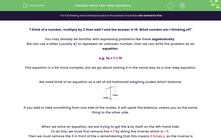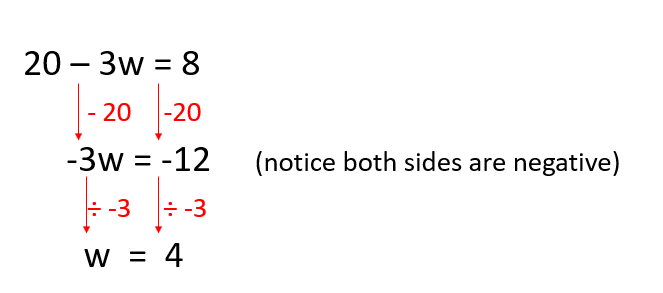# Solve Two-Step Equations

In this worksheet, students will learn how to solve two-step equations to find the value of a variable represented by a letter.Key stage:  KS 4

Year:  GCSE

GCSE Subjects:   Maths

GCSE Boards:   Pearson Edexcel, OCR, Eduqas, AQA,

Curriculum topic:   Algebra

Curriculum subtopic:   Solving Equations and Inequalities Algebraic Equations

Difficulty level:#### Worksheet Overview

"I think of a number, multiply by 3 then add 7 and the answer is 19. What number am I thinking of?"

You may already be familiar with expressing problems like these algebraically

We can use a letter (usually x) to represent an unknown number, then we can write the problem as an equation.

e.g. 3x + 7 = 19

This equation is a bit more complex, but we go about solving it in the same way as a one-step equation.

We need think of an equation as a set of old fashioned weighing scales which balance:If you add or take something from one side of the scales, it will upset the balance, unless you so the same thing to the other side.

When we solve an equation, we are trying to get the x by itself on the left-hand side.

To do this, we must first remove the + 7 by doing the inverse which is - 7

Then we must remove the 3 in front of the x remembering that this means 3 times x, so the inverse is divide bx y 3

We set this out like this:So the solution to this equation is: x = 4

Let's try another example now.

e.g. 8x - 7 = 9

Remember our scales, what we do to one side of the equation, we must do to the other.

Remember to do the inverse of -7 before the inverse of x8:Remember, x is not always used as the unknown, we could use any letter but the principle is still the same.

Here's one more example before it's over to you.

e.g. 20 - 3w = 8

Now, this is a little trickier as the 3w is being subtracted from the 20, not the other way round.

This is how we need to solve this equation:Remember if you divide a negative by a negative you get a positive.

Now it's over to you.

In this activity, we will solve two-step equations to find the value of a variable represented by a letter.

All the best!

### What is EdPlace?

We're your National Curriculum aligned online education content provider helping each child succeed in English, maths and science from year 1 to GCSE. With an EdPlace account you’ll be able to track and measure progress, helping each child achieve their best. We build confidence and attainment by personalising each child’s learning at a level that suits them.

Get started